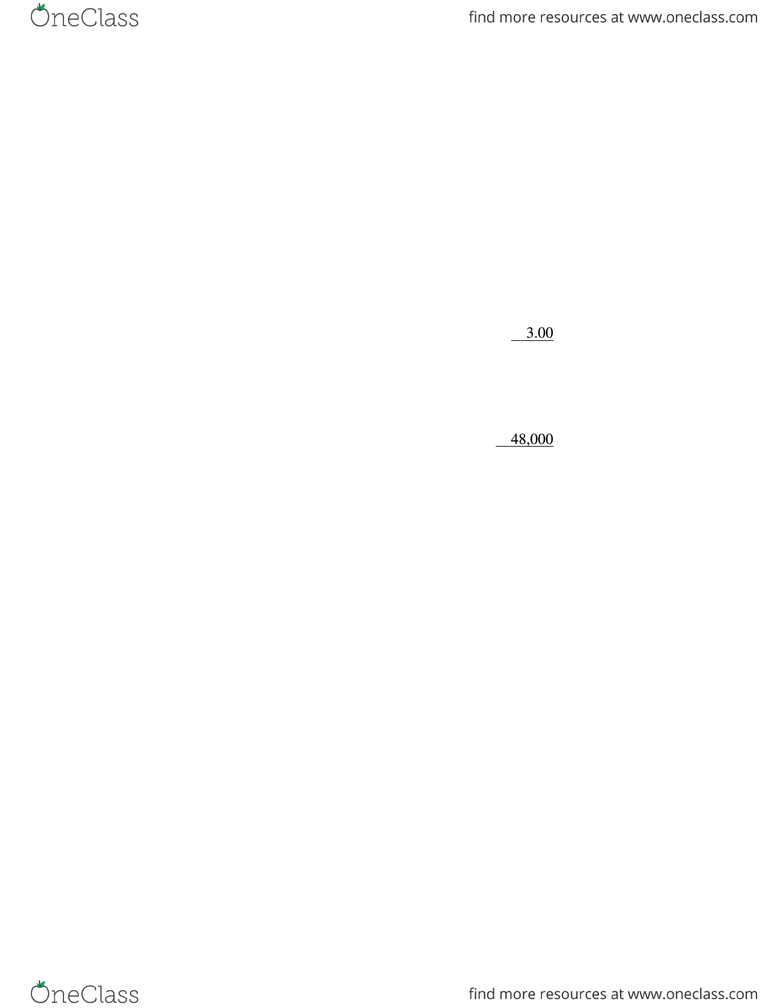Class Notes (1,000,000)
CA (620,000)
UTSC (30,000)
MGA (200)
Lecture 3

MGAB03H3 Lecture Notes - Lecture 3: Fixed Cost, Net Income

Department
Financial Accounting
Course Code
MGAB03H3
Professor
G.Quan Fun
Lecture
3

Page:
of 2Mastery Question
Maro and Company, a manufacturer of quality handmade walnut bowls, has experienced steady
growth in sales for the past five year. However, increased competition has led the President to
believe that an aggressive marketing campaign will be necessary next year to maintain the
company’s present growth.
To prepare for next year’s marketing campaign, the company’s controller has prepared and
presented Maro with the following date for the current year, 2011:
Variable costs (per bowl):
Direct labour \$ 9.60
Direct materials 3.90
Total variable costs \$16.50
Fixed cost:
Manufacturing \$ 30,000
Marketing and distribution 48,000
Total fixed cost \$162,000
Selling price per bowl \$30.00
Expected revenue, 2011 (20,000 units) \$600,000
Income tax rate 40%
Required:
1. What is the projected net income for 2011?
2. What is the breakeven point in units for 2011?
3. Maro has set the revenue target for 2012 at a level of \$660,000 (or 22,000 bowls). She
believes an additional marketing cost of \$13,500 for advertising in 2012, with all other costs
remaining constant, will be necessary to attain the revenue target. What will be the net
income for 2012 if the additional \$13,500 is spent and the revenue target is met?
4. What will be the breakeven point in revenues for 2012 if the additional \$13,500 is spent on
5. If the additional \$13,500 is spent for advertising in 2012, what is the required 2012 revenue
for 2012’s net income to equal 2011’s net income?
6. At a sales level of 22,000 units, what maximum amount can be spent on advertising if a 2012
net income of \$72,000 is desired?
Mastery Question- Solution
1. Revenues – Variable costs – Fixed costs = Target net income
1 – Tax rate
Let X = Net income for 2011
20,000(\$30.00) – 20,000(\$16.50) – \$162,000 = X
1 – 0.40
\$600,000 – \$330,000 – \$162,000 = X
0.60
(0.6)(\$108,000) = X
X = \$64,800
2. Let Q = Number of units to break even
\$30.00Q – \$16.50Q – \$162,000 = 0
Q = \$162,000 ÷ \$13.50 = 12,000 units
3. Let X = Net income for 2012
22,000(\$30.00) – 22,000(\$16.50) – (\$162,000 + \$13,500) = X
1 – 0.40
\$297,000 – \$175,500 = X
0.60
\$121,500 = X
0.60
X = \$72,900
4. Let Q = Number of units to break even with new fixed costs of \$175,500
\$30.00Q – \$16.50Q – \$175,500 = 0
Q = \$175,500 ÷ \$13.50 = 13,000 units
Revenues = 13,000(\$30.00) = \$390,000
Alternatively, the computation could be \$175,500 divided by the contribution margin percentage
of 45% to obtain \$390,000.
5. Let S = Required sales units to equal 2011 net income
\$30.00S – \$16.50S – \$175,500 = \$64,800
0.6
\$13.50S = \$283,500
S = 21,000 units
Revenues = 21,000 units × \$30.00 = \$630,000
6. Let A = Amount spent for advertising in 2012
\$660,000 – \$363,000 – (\$162,000 + A) = \$72,000
0.6
\$297,000 – \$162,000 – A = \$120,000
A = \$15,000